# 从辐角敏感场提取电学全息波传播轨迹Extracting the Propagating Trace of Electric Holographic Wave from the Argument Sensitivity Field

DOI: 10.12677/MP.2020.105011, PDF, HTML, XML, 下载: 21  浏览: 65

Abstract: The argument sensitivity field is a holographic sensitivity field expressed by the partial derivative of the electric holographic wave with respect to the argument. It is effective to use such a sensitivity field to extract the propagating trace of electric holographic wave with soft field effect under the working mode of single transmitting sensor and integrated receiving sensor with functions of receiving and grounding. If it is really necessary to adopt the multi-sensor transmitting mode, fine tuning on the parameters of transmitting sensors might be necessary.

1. 引言

2. 电学全息模型

2.1. 物理结构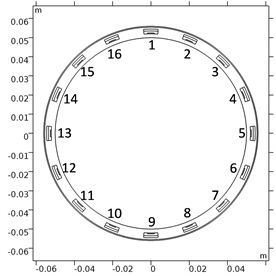Figure 1. Physical structure of electric holographic model

2.2. 传感器工作模式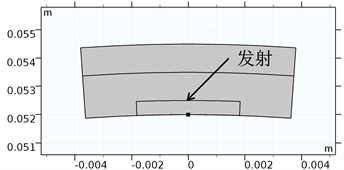(a)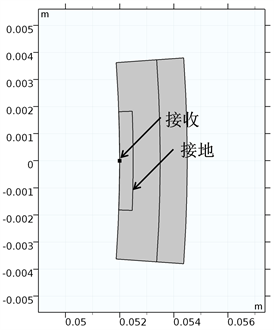(b)

Figure 2. Working mode of electric holographic sensor. (a) No. 1 sensor; (b) No. 5 sensor

2.3. 数学表达

$\left\{\begin{array}{l}\nabla \cdot \left(\epsilon \cdot \nabla \phi \right)=0,\text{\hspace{0.17em}}\text{\hspace{0.17em}}\phi \in D\\ \phi \left({\Gamma }_{T}\right)=1\\ \phi \left({\Gamma }_{E}\right)=0\end{array}$ (1)

3. 辐角敏感场

3.1. 数学表达

$\nabla \phi =\frac{\partial \phi }{\partial r}{e}_{r}+\frac{1}{r}\frac{\partial \phi }{\partial \theta }{e}_{\theta }$ (2)

${S}_{r}=\frac{\partial \phi }{\partial r}=\frac{x}{\sqrt{{x}^{2}+{y}^{2}}}\frac{\partial \phi }{\partial x}+\frac{y}{\sqrt{{x}^{2}+{y}^{2}}}\frac{\partial \phi }{\partial y}$ (3)

${S}_{\theta }=|\frac{1}{r}\frac{\partial \phi }{\partial \theta }|=|\frac{y}{\sqrt{{x}^{2}+{y}^{2}}}\frac{\partial \phi }{\partial x}-\frac{x}{\sqrt{{x}^{2}+{y}^{2}}}\frac{\partial \phi }{\partial y}|$ (4)

3.2. 初步试算

(1) 辐角敏感场在发射、接收传感器之间的劣弧周围具有较强的响应程度；

(2) 电学全息波传播轨迹通常为弧线而非总是为直线，深化了对电学全息波软场效应的认识；

(3) 辐角敏感场在电学全息波传播轨迹处的响应程度最弱，为提取电学全息波传播轨迹提供了依据。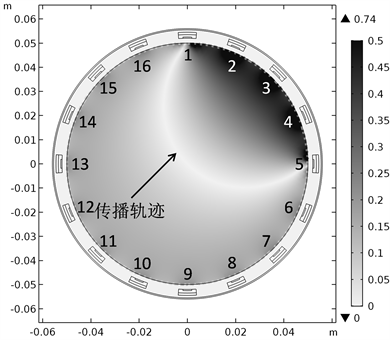Figure 3. Phase sensitivity field when No. 1 sensor transmits and No. 5 sensor receives

3.3. 轨迹提取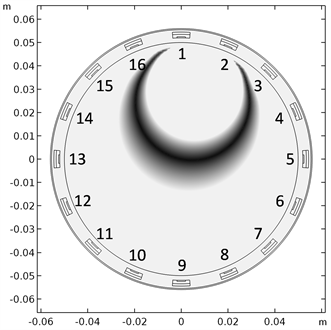(a)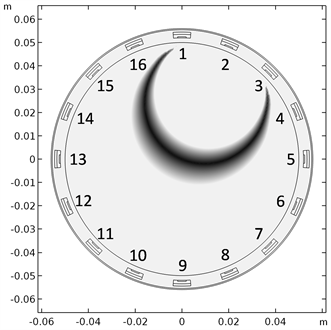(b)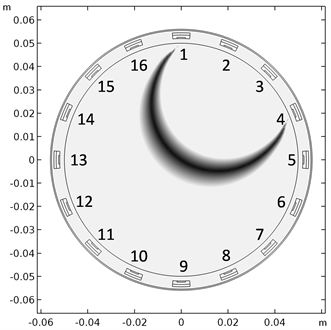(c)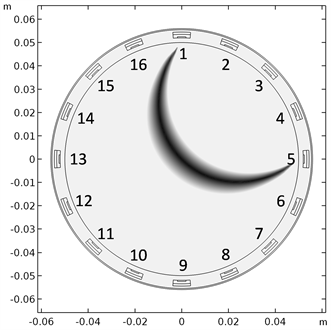(d)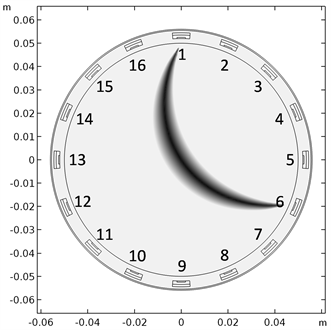(e)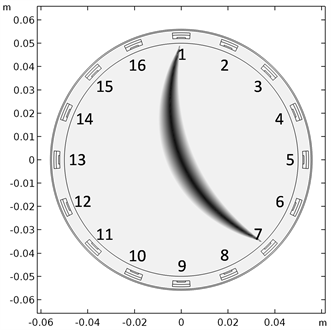(f)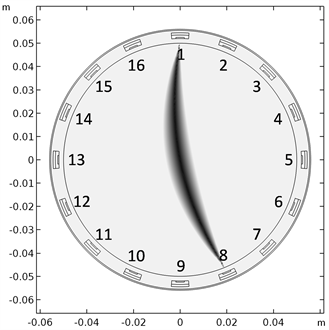(g)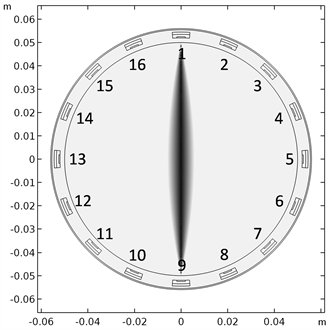(h)

Figure 4. Propagating traces of electric holographic wave when No. 1 sensor transmits and No. 2 - 9 sensors receive. (a) No. 2 sensor as the receiving sensor; (b) No. 3 sensor as the receiving sensor; (c) No. 4 sensor as the receiving sensor; (d) No. 5 sensor as the receiving sensor; (e) No. 6 sensor as the receiving sensor; (f) No. 7 sensor as the receiving sensor; (g) No. 8 sensor as the receiving sensor; (h) No. 9 sensor as the receiving sensor

4. 讨论

4.1. 局限性分析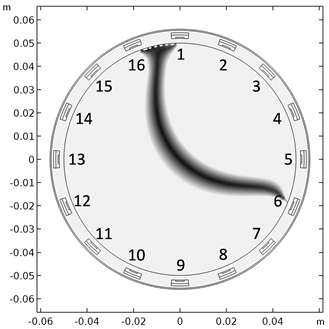(a)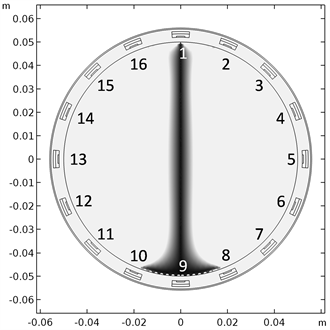(b)

Figure 5. Propagating traces of electric holographic wave when No. 1 sensor transmits and No. 5, 9 sensors receive under the previous sensor working condition. (a) No. 5 sensor as the receiving sensor; (b) No. 9 sensor as the receiving sensor

5号传感器接收为例，此时与发射传感器相邻的16号、2号传感器发射与1号传感器具有相同参数的电学全息波，作为聚焦传感器，与接收传感器相邻的4号、6号传感器作为接地传感器，也在一定程度上起到聚焦的作用。在这种工作模式下，5号、9号传感器接收时的电学全息波传播轨迹分别如图5(a)、图5(b)所示。分别与图4(d)、图4(f)进行对比可知，聚焦传感器和接地传感器的存在，虽然起到了场强增益的作用，但也使得电学全息波传播轨迹发生了偏离，可能会使实际的电学全息过程产生偏差。

4.2. 一种可能的解决方案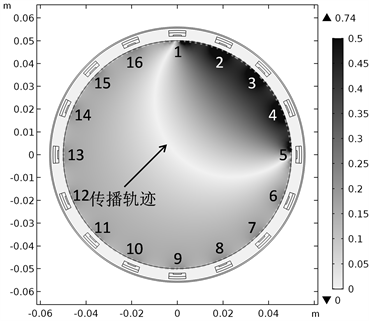Figure 6. Propagating traces of electric holographic wave when No. 1 sensor transmits and No. 5 sensor receives after applying a solution

5. 结论与引申

(1) 在发射传感器单一、接收传感器接收和接地功能合一的工作模式下，辐角敏感场能够用于提取具有软场效应的电学全息波传播轨迹。

(2) 采用多传感器发射模式时，对发射传感器参数进行微调，依然有望得到准确可靠的电学全息波传播轨迹。

(3) 毋庸置疑，采用辐角敏感场提取电学全息波传播轨迹的思想对非均匀介质分布下的电学全息过程依然适用。

  张阔, 吴锡令, 闫景富, 等. 油井多相流动电磁全息方法研究[J]. 石油科学通报, 2016, 1(2): 209-224.  张阔. 电学全息测量处理成像一体化软件架构设计[J]. 山东工业技术, 2020(2): 28-32.  张阔, 吴锡令, 蔡家铁. 油井流动成像电磁测量相位场特性[J]. 地球物理学进展, 2015, 30(4): 1843-1848.  Zhang, K., Wu, X.L., Yan, J.F., et al. (2017) Electromagnetic Holographic Sensitivity Field of Two-Phase Flow in Horizontal Wells. Applied Geophysics, 14, 40-48. https://doi.org/10.1007/s11770-017-0606-0  张阔, 吴锡令, 闫景富, 等. 油井电磁全息成像精度和分辨率初探[J]. 地球物理学进展, 2019, 34(1): 251-256.  张阔. 电学全息传感器智能化测量设计及应用[J]. 新一代信息技术, 2019, 2(14): 12-16.  Zhang, K., Wu, X., Yan, J., et al. (2017) An Electric-Based Dual-Mode Tomography System for Interpretation on Two-Phase Flow in Oil Wells. Interpretation, 5, T259-T264. https://doi.org/10.1190/INT-2016-0215.1  张阔. 电极不一致性对电学全息测量的影响[J]. 新一代信息技术, 2019, 2(23): 9-17.